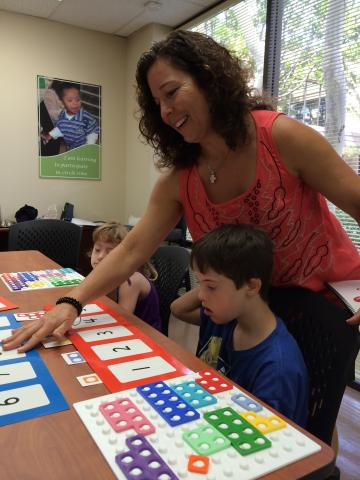October 2015 – This month Pre-K and Level 1 sessions focus on the early number concepts of rote counting, number recognition and counting with meaning. Spending time on these concepts helps students build a solid math foundation which will be useful as they work on higher level math skills such as adding, subtracting, time and money. Initial goals are to rote count, identify numbers and count with meaning to 5, 10, 20 and above.

Level 2 students review early number concepts, but also focus on learning about place value and subitizing. Place value is important once students add numbers greater than 5 or for solving multi-digit equations.

Last, but not least, our Level 3 and 4 classes focus on adding and subtracting with objects, fingers, drawings and formal equations. As we work with students in the upper levels, we are mindful of their need to understand both “what” and “how” (the concept AND the calculation). Getting the correct answer without understanding the concept isn’t helpful in the long term.  Extra time is dedicated to strategies for solving word problems, multi-digit equations and solving for the unknown.